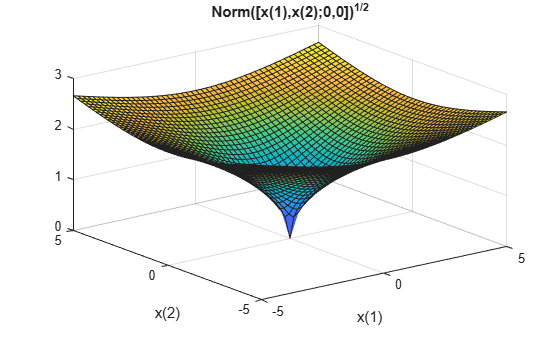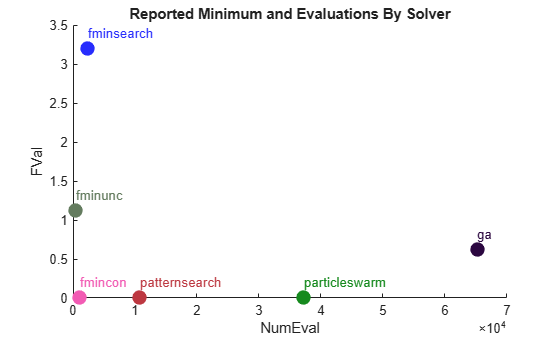Documentation

### This is machine translation

Mouseover text to see original. Click the button below to return to the English version of the page.

## Solver Behavior with a Nonsmooth Problem

This example shows the importance of choosing an appropriate solver for optimization problems. It also shows that a single point of non-smoothness can cause problems for Optimization Toolbox™ solvers.

In general, the solver decision tables provide guidance on which solver is likely to work best for your problem. For smooth problems, see Optimization Decision Table (Optimization Toolbox). For nonsmooth problems, see Table for Choosing a Solver first, and for more information consult Global Optimization Toolbox Solver Characteristics.

### A Function with a Single Nonsmooth Point

The function $f\left(x\right)=||x|{|}^{1/2}$ is nonsmooth at the point 0, which is the minimizing point. Here is a 2-D plot using the matrix norm for the 4-D point $\left[\begin{array}{cc}x\left(1\right)& x\left(2\right)\\ 0& 0\end{array}\right]$ .

```figure x = linspace(-5,5,51); [xx,yy] = meshgrid(x); zz = zeros(size(xx)); for ii = 1:length(x) for jj = 1:length(x) zz(ii,jj) = sqrt(norm([xx(ii,jj),yy(ii,jj);0,0])); end end surf(xx,yy,zz) xlabel('x(1)') ylabel('x(2)') title('Norm([x(1),x(2);0,0])^{1/2}')```This example uses matrix norm for a 2-by-6 matrix `x`. The matrix norm relates to the singular value decomposition, which is not as smooth as the Euclidean norm. See 2-Norm of Matrix (MATLAB).

### Minimize Using `patternsearch`

`patternsearch` is the recommended first solver to try for nonsmooth problems. See Table for Choosing a Solver. Start `patternsearch` from a nonzero 2-by-6 matrix `x0`, and attempt to locate the minimum of $f$. For this attempt, and all others, use the default solver options.

Return the solution, which should be near zero, the objective function value, which should likewise be near zero, and the number of function evaluations taken.

```fun = @(x)norm([x(1:6);x(7:12)])^(1/2); x0 = [1:6;7:12]; rng default x0 = x0 + rand(size(x0))```
```x0 = 2×6 1.8147 2.1270 3.6324 4.2785 5.9575 6.1576 7.9058 8.9134 9.0975 10.5469 11.9649 12.9706 ```
`[xps,fvalps,eflagps,outputps] = patternsearch(fun,x0);`
```Optimization terminated: mesh size less than options.MeshTolerance. ```
`xps,fvalps,eflagps,outputps.funccount`
```xps = 2×6 10-4 × 0.1116 -0.1209 0.3503 -0.0520 -0.1270 0.2031 -0.3082 -0.1526 0.0623 0.0652 0.4479 0.1173 ```
```fvalps = 0.0073 ```
```eflagps = 1 ```
```ans = 10780 ```

`patternsearch` reaches a good solution, as evinced by exit flag 1. However, it takes over 10,000 function evaluations to converge.

### Minimize Using `fminsearch`

The documentation states that `fminsearch` sometimes can handle discontinuities, so this is a reasonable option.

`[xfms,fvalfms,eflagfms,outputfms] = fminsearch(fun,x0);`
``` Exiting: Maximum number of function evaluations has been exceeded - increase MaxFunEvals option. Current function value: 3.197063 ```
`xfms,fvalfms,eflagfms,outputfms.funcCount`
```xfms = 2×6 2.2640 1.1747 9.0693 8.1652 1.7367 -1.2958 3.7456 1.2694 0.2714 -3.7942 3.8714 1.9290 ```
```fvalfms = 3.1971 ```
```eflagfms = 0 ```
```ans = 2401 ```

Using default options, `fminsearch` runs out of function evaluations before it converges to a solution. Exit flag 0 indicates this lack of convergence. The reported solution is poor.

### Use `particleswarm`

`particleswarm` is recommended as the next solver to try. See Choosing Between Solvers for Nonsmooth Problems.

`[xpsw,fvalpsw,eflagpsw,outputpsw] = particleswarm(fun,12);`
```Optimization ended: relative change in the objective value over the last OPTIONS.MaxStallIterations iterations is less than OPTIONS.FunctionTolerance. ```
`xpsw,fvalpsw,eflagpsw,outputpsw.funccount`
```xpsw = 1×12 10-12 × -0.0386 -0.1282 -0.0560 0.0904 0.0771 -0.0541 -0.1189 0.1290 -0.0032 0.0165 0.0728 -0.0026 ```
```fvalpsw = 4.5222e-07 ```
```eflagpsw = 1 ```
```ans = 37200 ```

`particleswarm` finds an even more accurate solution than `patternsearch`, but takes over 35,000 function evaluations. Exit flag 1 indicates that the solution is good.

### Use `ga`

`ga` is a popular solver, but is not recommended as the first solver to try. See how well it works on this problem.

`[xga,fvalga,eflagga,outputga] = ga(fun,12);`
```Optimization terminated: average change in the fitness value less than options.FunctionTolerance. ```
`xga,fvalga,eflagga,outputga.funccount`
```xga = 1×12 -0.0061 -0.0904 0.0816 -0.0484 0.0799 -0.1925 0.0048 0.3581 0.0848 0.0232 0.0237 -0.1294 ```
```fvalga = 0.6257 ```
```eflagga = 1 ```
```ans = 68600 ```

`ga` does not find as good a solution as `patternsearch` or `particleswarm`, and takes about twice as many function evaluations as `particleswarm`. Exit flag 1 is misleading in this case.

### Use `fminunc` from Optimization Toolbox

`fminunc` is not recommended for nonsmooth functions. See how it performs on this one.

`[xfmu,fvalfmu,eflagfmu,outputfmu] = fminunc(fun,x0);`
```Local minimum possible. fminunc stopped because the size of the current step is less than the value of the step size tolerance. ```
`xfmu,fvalfmu,eflagfmu,outputfmu.funcCount`
```xfmu = 2×6 -0.5844 -0.9726 -0.4356 0.1467 0.3263 -0.1002 -0.0769 -0.1092 -0.3429 -0.6856 -0.7609 -0.6524 ```
```fvalfmu = 1.1269 ```
```eflagfmu = 2 ```
```ans = 442 ```

The `fminunc` solution is not as good as the `ga` solution. However, `fminunc` reaches the rather poor solution in relatively few function evaluations. Exit flag 2 means you should take care, the first-order optimality conditions are not met at the reported solution.

### Use `fmincon` from Optimization Toolbox

`fmincon` can sometimes minimize nonsmooth functions. See how it performs on this one.

`[xfmc,fvalfmc,eflagfmc,outputfmc] = fmincon(fun,x0);`
```Local minimum possible. Constraints satisfied. fmincon stopped because the size of the current step is less than the value of the step size tolerance and constraints are satisfied to within the value of the constraint tolerance. ```
`xfmc,fvalfmc,eflagfmc,outputfmc.funcCount`
```xfmc = 2×6 10-10 × 0.2138 0.4892 0.0446 0.3698 0.1195 0.1112 0.4987 0.4086 -0.5749 0.1132 0.4341 0.2668 ```
```fvalfmc = 1.0258e-05 ```
```eflagfmc = 2 ```
```ans = 1052 ```

`fmincon` with default options produces an accurate solution after fewer than 1000 function evaluations. Exit flag 2 does not mean that the solution is inaccurate, but that the first-order optimality conditions are not met. This is because the gradient of the objective function is not zero at the solution.

### Summary of Results

Choosing the appropriate solver leads to better, faster results. This summary shows how disparate the results can be. The solution quality is `'Poor'` if the objective function value is greater than 0.1, `'Good'` if the value is smaller than 0.01, and `'Mediocre'` otherwise.

```Solver = {'patternsearch';'fminsearch';'particleswarm';'ga';'fminunc';'fmincon'}; SolutionQuality = {'Good';'Poor';'Good';'Poor';'Poor';'Good'}; FVal = [fvalps,fvalfms,fvalpsw,fvalga,fvalfmu,fvalfmc]'; NumEval = [outputps.funccount,outputfms.funcCount,outputpsw.funccount,... outputga.funccount,outputfmu.funcCount,outputfmc.funcCount]'; results = table(Solver,SolutionQuality,FVal,NumEval)```
```results=6×4 table Solver SolutionQuality FVal NumEval _______________ _______________ __________ _______ 'patternsearch' 'Good' 0.0072656 10780 'fminsearch' 'Poor' 3.1971 2401 'particleswarm' 'Good' 4.5222e-07 37200 'ga' 'Poor' 0.62572 68600 'fminunc' 'Poor' 1.1269 442 'fmincon' 'Good' 1.0258e-05 1052 ```

Another view of the results.

```figure hold on for ii = 1:length(FVal) clr = rand(1,3); plot(NumEval(ii),FVal(ii),'o','MarkerSize',10,'MarkerEdgeColor',clr,'MarkerFaceColor',clr) text(NumEval(ii),FVal(ii)+0.2,Solver{ii},'Color',clr); end ylabel('FVal') xlabel('NumEval') title('Reported Minimum and Evaluations By Solver') hold off```While `particleswarm` achieves the lowest objective function value, it does so by taking over three times as many function evaluations as `patternsearch`, and over 30 times as many as `fmincon`.

`fmincon` is not generally recommended for nonsmooth problems. It is effective in this case, but this case has just one nonsmooth point.

Watch now